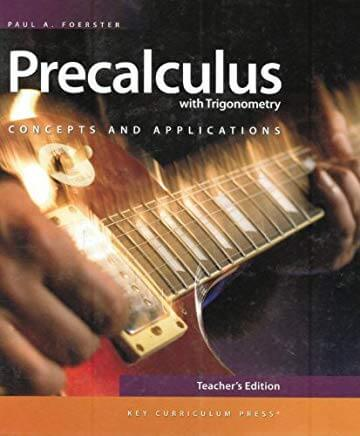# Foerster’s Precalculus with Trigonometry: Student Edition

\$153.55

Paul A. Foerster has taught mathematics at Alamo Heights High School in San Antonio, Texas since 1961. In that same year he received his teaching certificate from Texas A&M University. His B.S. degree in Chemical Engineering and M.A. degree in Mathematics are from the University of Texas. Among many honors, he was awarded the Presidential Award for Excellence in Mathematics Teaching in 1983. He brings to his teaching and textbook writing the insights from his engineering background. His textbooks contain some of the best collections of real-world applications to be found in any algebra/calculus textbooks.

This Precalculus course is an advanced math course that combines the study of trigonometry, functions, analytic geometry, and math analysis topics as preparation for calculus. Topics include the study of complex numbers; polynomial, logarithmic, exponential, rational, trigonometric, and circular functions, including their relations; inverses and graphs; trigonometric identities and equations; solutions of right and oblique triangles; vectors; the polar coordinate system; conic sections; symbolic logic; mathematical induction; matrix algebra; sequences and series; limits and continuity.

A strong emphasis is placed on critical thinking skills and written communication of methods for problem solving. The intent is to develop a deep conceptual understanding of each topic, making connections to other areas and topics in mathematics and to develop strong problem-solving skills. Students are asked to explain answers and show methods, not just state answers.

Cathy Duffy says, “Foerster’s books, in particular, have long been recognized as among the best high school math texts.”